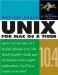# Doing Arithmetic and Using Expressions

Doing Arithmetic and Using Expressions

It is frequently useful to have a script add two numbers together, or perform multiplication, division, or other arithmetic. The Bourne shell itself does not provide built-in arithmetic functions. Instead, it uses the standard Unix expr command to evaluate expressions .

The expr command sees expressions as a set of three arguments consisting of two arguments with an operator between them, such as

3 + 4

or

\$x \$y

Table 9.2 lists the operators you are most likely to use with expr . Read the man page ( man expr ) for the complete list. The math operators supported by expr work only on integers and give only integers as resultsno decimal places. See the sidebar "Floating-Point Math" for notes about doing this type of calculation.

##### Table 9.2. Math Operators for Use with expr

All numerical values must be integers. See the expr man page for other operators.

E XPRESSION

M EANING

\$a + \$b

Returns the sum of \$a and \$b .

\$a - \$b

Subtracts \$b from \$a .

\$a \* \$b

Returns the product of \$a times \$b . (You must escape the * with a backslash.)

\$a / \$b

Divides \$a by \$b .

\$a % \$b

Returns the remainder after dividing \$a by \$b .

Try the following task at a shell prompt before you try it in a script.

To evaluate an expression:

• expr 3 + 4

This should give you a result of 7. The spaces before and after the + are required, as expr needs to see each item as a separate argument ( 3 , + , and 4 ).

Figure 9.18 is a code listing that shows a script using the expr command in backquotes to perform integer arithmetic, and Figure 9.19 shows the output of the script.

##### Figure 9.18. Code listing of a script that uses the expr command in backquotes to perform integer arithmetic.
` #!/bin/sh # This script uses expr to perform integer arithmetic. total=`expr  + ` difference=`expr  - ` product=`expr  \* ` fraction=`expr  / ` remainder=`expr  % ` echo "\$total is the sum of  and " echo "\$difference is the difference between  and " echo "\$product is the product of  and " echo "\$fraction is  divided by " echo "\$remainder is the remainder of dividing  by " `

##### Figure 9.19. Output from the script in Figure 9.18.
` localhost:~ vanilla\$  ./math.sh 517 23  540 is the sum of 517 and 23 494 is the difference between 517 and 23 11891 is the product of 517 and 23 22 is 517 divided by 23 11 is the remainder of dividing 517 by 23 [localhost:~] `

To add something to an existing variable:

• Simply use the variable as one of the arguments to expr .

Let's say you want to add 1 to whatever is stored in \$count . You could use this:

count=`expr \$count + 1`

This construct is often used inside loops to count the number of times a loop was executed. See "Using loops ," later in this chapter.Unix for Mac OS X 10.4 Tiger: Visual QuickPro Guide (2nd Edition)
ISBN: 0321246683
EAN: 2147483647
Year: 2004
Pages: 161
Authors: Matisse Enzer

Similar book on Amazon